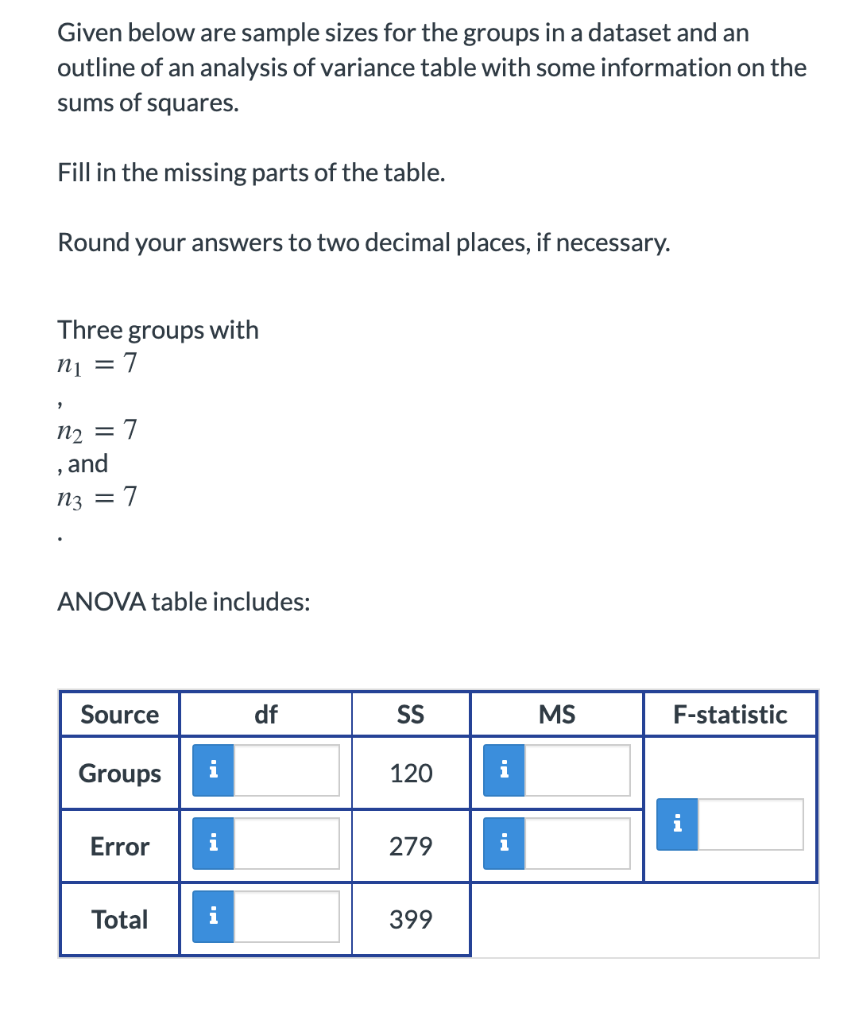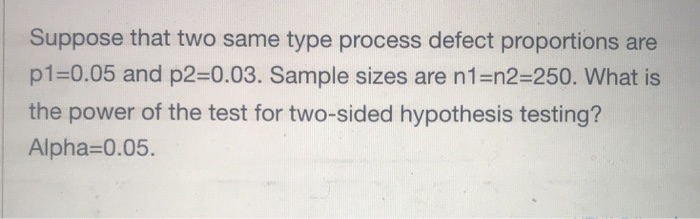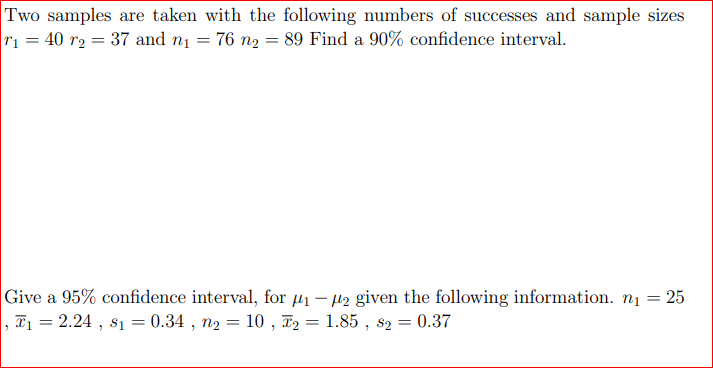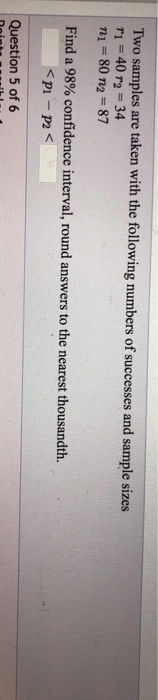Question

# show work pleas Given the following sample sizes, determine the df. n1 = 18 and n2...

show work pleas
Given the following sample sizes, determine the df. n1 = 18 and n2 = 22

3. Given the following sample sizes, determine the df. n1 = 40 and n2 = 40

n1 = 18, n2 = 22
df = ?
df = n1 + n2 - 2
df = 18 + 22 - 2
df = 40 - 2
df = 38

3) n1 = 40, n2 = 40
df = ?
df = n1 + n2 - 2
df = 40 + 40 - 2
df = 80 - 2
df = 78

#### Earn Coins

Coins can be redeemed for fabulous gifts.

Similar Homework Help Questions
• ### 10. If we are doing two-sample hypothesis testing with related samples of sizes n1 = 10...

10. If we are doing two-sample hypothesis testing with related samples of sizes n1 = 10 and n2 = 10, what is the number of degrees of freedom? a. 9 b. 10 c. 19 d. 20 11. If we are doing two-sample hypothesis testing with independent samples of sizes n1 = 10 and n2 = 10, what is the number of degrees of freedom? a. 10 b. 18 c. 19 d. 20

• ### Given below are sample sizes for the groups in a dataset and an outline of an...Given below are sample sizes for the groups in a dataset and an outline of an analysis of variance table with some information on the sums of squares. Given below are sample sizes for the groups in a dataset and an outline of an analysis of variance table with some information on the sums of squares. Fill in the missing parts of the table. Round your answers to two decimal places, if necessary. Three groups with n1 = 7 n2...

• ### Two samples are taken with the following sample means, sizes, and standard deviations ¯ x1 x¯1...

Two samples are taken with the following sample means, sizes, and standard deviations ¯ x1 x¯1 = 31 ¯ x2 x¯2 = 28 n1 n1 = 70 n2 n2 = 46 s1 s1 = 4 s2 s2 = 2 We want to estimate the difference in population means using a 89% confidence level. What distribution does this require? t, df = 108 z NOTE: The more accurate df formula, used above, is: df= ( s 2 1 n1 + s...

• ### Suppose that independent samples of sizes n1, n2, . . . , nk are taken from each of k normally di...

Suppose that independent samples of sizes n1, n2, . . . , nk are taken from each of k normally distributed populations with means μ1,μ2, . . . , μk and common variances, all equal to σ 2. Let Yi j denote the j th observation from population i, for j = 1, 2, . . . , ni and i = 1, 2, . . . , k, and let n = n1 + n2 + ··· + nk...

• ### Suppose that two same type process defect proportions are p1=0.05 and p2=0.03. Sample sizes are n1=n2-250....Suppose that two same type process defect proportions are p1=0.05 and p2=0.03. Sample sizes are n1=n2-250. What is the power of the test for two-sided hypothesis testing? Alpha-0.05.

• ### a) b) Two samples are taken with the following numbers of successes and sample sizes r1...a) b) Two samples are taken with the following numbers of successes and sample sizes r1 = 40 r2 = 37 and n1 = 76 n2 = 89 Find a 90% confidence interval. Give a 95% confidence interval, for H1 - H2 given the following information. Ni = 25 , T1 = 2.24 , \$i = 0.34 , n2 = 10 , T2 = 1.85 , \$2 = 0.37

• ### Two samples are taken with the following numbers of successes and sample sizes

Two samples are taken with the following numbers of successes and sample sizesr1 = 40 r2 = 32n1 = 69 n2 = 85Find a 91% confidence interval, round answers to the nearest thousandth.___ < p1 - p2 < ___

• ### The numbers of successes and the sample sizes are given for independent simple random samples from...

The numbers of successes and the sample sizes are given for independent simple random samples from two populations. Use the two-proportions z-test to conduct the required hypothesis test. Use the critical-value approach. x1 = 24, n1 = 60, x2 = 12, n2 = 40, two-tailed test, α = 0.05

• ### The numbers of successes and the sample sizes are given for independent simple random samples from...

The numbers of successes and the sample sizes are given for independent simple random samples from two populations. Use the two-proportions z-test to conduct the required hypothesis test. Use the critical-value approach. x1 = 24, n1 = 60, x2 = 28, n2 = 40, left-tailed test, α = 0.05

• ### Two samples are taken with the following numbers of successes and sample sizes 1 40 r2...Two samples are taken with the following numbers of successes and sample sizes 1 40 r2 34 n1 80 n2-87 Find a 98% confidence interval, round answers to the nearest thousandth. p1 -p2 Question 5 of 6